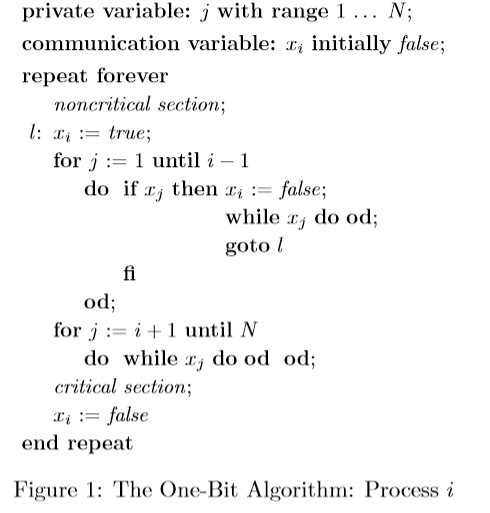## Friday, June 5, 2015

### Lamport's One bit solution in C11

As we mentioned before, Lamport's One Bit Solution is one of the fastest (if not the fastest) software solution to the mutual exclusion problem that uses only loads and stores.
Here is the original algorithm:Andreia came up with an almost identical solution earlier this year, having only a small variation on the "backoff strategy", which in Lamport's case is spinning on each entry of the array until all have been traversed, while on Andreia's variant the waiting thread just starts over. This gives a (small) statistical advantage so that the threads don't trample over each other, but the basic idea of the algorithm is the same: threads to the right of your entry in the array have priority over you, and you have priority over the threads to the left.
What this implies is that the thread with entry zero in the array has priority over all other threads and can easily starve them. This algorithm is not starvation-free (if you want to see a variation of this algorithm that is starvation-free, then check out CRTurn in our paper).

Here's what Andreia's variant looks like in C11 using memory_order_seq_cst:
while (1) {
int st = UNLOCKED;
for ( int j = 0; j < id; j += 1 )
if ( (st = atomic_load(&states[j*PADRATIO])) == LOCKED ) break;
if (st == LOCKED) {
while (1) {
for ( int j = 0; j < N; j += 1 )
if ( (st = atomic_load(&states[j*PADRATIO])) == LOCKED ) break;
if (st == UNLOCKED) break;
Pause();
}
continue;
}
}
for ( int j = id + 1; j < N; j += 1 )
while (atomic_load(&states[j*PADRATIO]) == LOCKED) { Pause(); }
CriticalSection( id );

where states[] is an array of atomic_int with N*PADRATIO entries (the maximum number of threads), id is the entry in states[] for the current thread, and PADRATIO is the size of a cache line (in atomic_ints).

In this implementation we are using only sequentially consistent atomics, and this is fine and correct, but it isn't terribly fast. Can we relax some of the atomic operations and still provide a correct algorithm?
Yes!

The most obvious improvement is to change the last atomic_store() to use memory_order_release. This will have no effect on the algorithm itself, because none of the instructions on Critical Section (CS) will be re-ordered with the atomic_store() seen as they are above it. Even when running in a loop, it will not be re-ordered with the first atomic_store() because atomic_stores() with memory_order_release will not be re-ordered.
This may seem like a small improvement, but on x86 it means we've just saved an MFENCE instruction, which improves performance dramatically, at least for the low contention scenario:The table above was taken from this PhD thesis, which I recommend if you want to do a deep dive into the C11/C++1x memory model.

A second optimization that does not change the algorithm, is to replace the sequentially consistent loads in the while() loop before the CS, with memory_order_acquire. Loads with a memory_order_acquire will never be re-ordered with each other or with any instructions below them, which means that they will be "stuck in place", and no instruction of the CS can be re-ordered with these loads.
Notice that they could be re-ordered with an atomic_store() immediately above them, but there is always at least one sequentially consistent atomic_load() above them (either from the first for() loop or from the second for() loop), and above those, the atomic_store() is also sequentially consistent (either the first atomic_store() or the second atomic_store()), and because atomic_stores() and atomic_loads() using memory_order_seq_cst can not be re-ordered, everything is safe and correct.
As you can see from the table above, this optimization will make no difference whatsoever on x86, but on PowerPC and ARM, it will save an hwsync or a dmb, both expensive instructions.

There is one more optimization we could do, but in practice it doesn't save much, so maybe I'll talk about some other time.

Here's what the optimized code looks like:

while (1) {
int st = UNLOCKED;
for ( int j = 0; j < id; j += 1 )
if ( (st = atomic_load(&states[j*PADRATIO])) == LOCKED ) break;
if (st == LOCKED) {
while (1) {
for ( int j = 0; j < N; j += 1 )
if ( (st = atomic_load(&states[j*PADRATIO])) == LOCKED ) break;
if (st == UNLOCKED) break;
Pause();
}
continue;
}
}
for ( int j = id + 1; j < N; j += 1 )
while (atomic_load_explicit(&states[j*PADRATIO], memory_order_acquire) == LOCKED) { Pause(); }
CriticalSection( id );